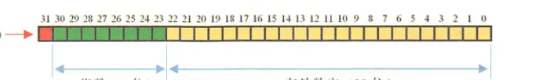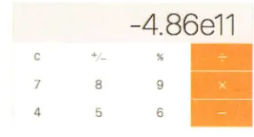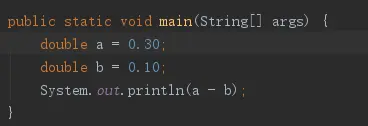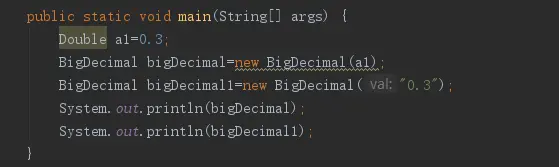# Why do double floating point operations lose precision?

## 1. What is a floating point number?

A floating point number is a type of data used by computers to represent decimals, using scientific notation. In java, double is a double-precision, 64-bit, floating-point number, and the default is 0.0d. float is a single-precision, 32-bit floating-point numbers, default is 0.0f.

Storing in memoryfloat sign bit (1 bit) exponent (8 bit) mantissa (23 bit) double sign bit (1 bit) exponent (11 bit) mantissa (52 bit)

The float exponent is 8bit in memory, and since the factorial actually stores the shift of the exponent, assuming that the true value of the exponent is e and the factorial is E, we have E=e+(2^n-1 - 1). Where 2^n-1 - 1 is the exponent offset specified by IEEE754 standard, according to this formula we can get 2^8 - 1=127. Thus, the exponent range of float is - 128 + 127, while the exponent range of double is - 1024 + 1023. where the negative exponent determines the smallest absolute non-zero number that can be expressed by a floating point number; while the positive exponent determines the absolute value that can be expressed by a The negative exponent determines the non-zero number with the smallest absolute value that can be expressed by a floating point number, while the positive exponent determines the number with the largest absolute value that can be expressed by a floating point number.

The range of float is - 2^128 ~ +2^127, i.e. - 3.40E+38 ~ +3.40E+38. The range of double is - 2^1024 ~ +2^1023, which is - 1.79E+308 ~ +1.79E+308

## 2. Into the Distortion of Scientific Notation

Let’s talk about scientific notation first. Scientific notation is a simplified counting method used to approximate a very large or very small number with a large number of digits, for small values, scientific notation has no advantage, but for values with a large number of digits the advantage of its counting method is very obvious. For example, the speed of light is 300,000,000 meters per second, and the world’s population is about 6,100,000,000. It is difficult to read and write large numbers like the speed of light and the world’s population, so the speed of light can be written as 310^8, and the world’s population can be written as 6.110^9. The population is about 6.1E9.

When we played with calculators as kids, we liked to add and subtract like crazy, and at the end of the day, the calculator would display the graph below. This is the result of scientific notation.The real value in that graph is -4.86*10^11=-486000000000. Decimal scientific notation requires that the integer part of a valid number must be in the [1, 9] range.

## 3. Into the accuracy of distortion

Computer in processing data are involved in the conversion of data and a variety of complex operations, such as, different units conversion, different decimal (such as binary decimal) conversion, etc., many division operations can not divide the exhaustive, such as 10 ÷ 3 = 3.3333 ….. infinite, and the precision is limited, 3.3333333x3 is not equal to 10, the decimal data obtained after complex processing is not precise, the higher the precision the more precise. float and double precision is determined by the number of bits in the tail, its integer part is always an implied “1 Since it is invariant, it cannot have an impact on precision. float: 2^23 = 8388608, seven bits in total, and since the leftmost bit is omitted for 1, this means that at most 8 bits can be represented: 2*8388608 = 16777216. There are 8 significant digits, but the absolute guarantee is 7, i.e.float has a precision of 7~8 significant digits; double: 2^52 = 4503599627370496, 16 bits in total, similarly, double has a precision of 16~17 bits.

Scientific notation is automatically started when a certain value is reached, and the valid digits of the relevant precision are retained, so the result is an approximation, and the exponent is an integer. Some of the decimal numbers in decimal cannot be represented completely in binary. Therefore, they can only be expressed in finite bits, and thus may have errors in storage. For decimal decimal to binary conversion, the multiplication by 2 method is used, where the integer part is removed and the remaining decimals are multiplied by 2 until the decimal part is all 0.

If you encounterthe output is 0.1999999999999999998

The case of double type 0.3-0.1. You need to convert 0.3 to binary in the operation

0.3 * 2 = 0.6 => .0 (.6) takes 0 and leaves 0.6 0.6 * 2 = 1.2 => .01 (.2) takes 1 and leaves 0.2 0.2 * 2 = 0.4 => .010 (.4) Take 0 and leave 0.4 0.4 * 2 = 0.8 => .0100 (.8) Take 0 and leave 0.8 0.8 * 2 = 1.6 => .01001 (.6) Take 1 and leave 0.6 ………….

## 3. Summary

After reading the above, it’s probably clear why floating point numbers have precision problems. Simply put, float and double types are designed primarily for scientific and engineering computations, and they perform binary floating-point operations, which are carefully designed to provide fast and relatively accurate approximations over a wide range of values. However, they do not provide completely accurate results, so they should not be used in situations where exact results are possible. Floating point numbers up to a certain size automatically use scientific notation, and such a representation only approximates the true number and is not equal to it. Infinite loops or exceeding the length of the floating point trailing number can also occur when decimal fractional bits are converted to binary.

## 4. So how do we solve it with BigDecimal?

Look at the following two outputsThe output is:

0.2999999999999999998889776975374843454595763683319091796875 0.3

Ari’s code constraint plugin on the chart has marked a warning to create BigDecimal using constructor method with String parameter. because double cannot be represented exactly as 0.3 (any finite length binary) and the value passed by constructor method is not exactly equal to 0.3. Everyone must create BigDecimal using constructor method with String parameter constructor. Speaking of this, is there any curious baby who has a question, what is the principle of BigDecimal? Why is there no problem with it? In fact, the principle is very simple, BigDecimal is immutable, and it can be used to represent the signed decimal number with any precision. double has a problem because the decimal point is lost to binary precision. **BigDecimal expands the decimal decimal by a factor of N to make it compute on the integer, and keeps the corresponding precision information when processing.**You can read the source code to see how BigDecimal is saved.